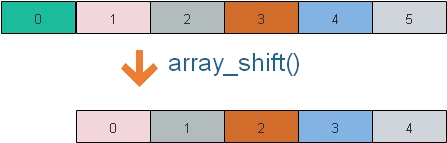# array_shift(): Taking out first element of the array

``\$output=array_shift(\$input);``Take out the first element of the array and returns the same. After applying `array_shift()` total elements of the array reduces by one. All numerical array keys are re-indexed but literal keys remain same.

Returns the shifted element ( first element ) of the array.

Here \$input is an array, \$output get the value of first element of the array.
``````<?Php
\$input=array('One','Two','Three','Four','Five');
\$output=array_shift(\$input);
echo \$output; // Output is  One
echo "<br><br>";
while (list (\$key, \$val) = each (\$input)) {
echo "\$key -> \$val <br>";
}
?>``````
Output of above code is here( \$output gets the first element One )
``````One

0 -> Two
1 -> Three
2 -> Four
3 -> Five ``````

## With Literal Keys

``````\$input=array('Fruits1' =>'Banana','Fruits2'=>'Mango','Fruits3'=>'Apple','Fruits4'=>'Grapes');
\$output=array_shift(\$input);
echo \$output; // Output is  Banana
echo "<br><br>";
while (list (\$key, \$val) = each (\$input)) {
echo "\$key -> \$val <br>";
}``````
Output is here, ( \$output gets the first element Banana)
``````Banana

Fruits2 -> Mango
Fruits3 -> Apple
Fruits4 -> Grapes``````

## Using current

By using current() we can get the element to which the present internal pointer is pointing. By default it points to first element of the array.
``````\$input=array('Fruits1' =>'Banana','Fruits2'=>'Mango','Fruits3'=>'Apple','Fruits4'=>'Grapes');
echo current(\$input);
echo "<br>";
\$output=array_shift(\$input);
echo current(\$input);``````
Output is here.( The first element is changed from Banana to Mango)
``````Banana
Mango``````Subscribe to our YouTube Channel here

## Subscribe

* indicates required
Subscribe to plus2netplus2net.com

Post your comments , suggestion , error , requirements etc here .